# Inclusive disjunction facts for kids

Kids Encyclopedia Facts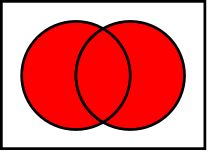Venn diagram of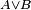$A \or B$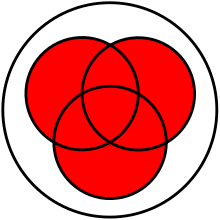Venn diagram of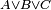$A \or B \or C$

Inclusive disjunction (also called or) is a logic operation. It normally takes two truth values as inputs and returns one truth value as output. It is false when both inputs are false, but is true otherwise. It is written with the symbol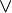$\lor$.

In general, given two propositions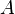$A$ and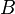$B$,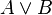$A \lor B$ is true if$A$ is true, or if$B$ is true, or if both$A$ and$B$ are true.

This is different from the exclusive disjunction, which asserts "either x or y, but not both".

## Related pagesInclusive disjunction Facts for Kids. Kiddle Encyclopedia.